# C033 – Density of Moist Air

## Description

This calculates the density of moist air from temperature, pressure, carbon dioxide content and one other parameter depending on the option selected.

Revised formula for the density of moist air (CIPM-2007) – A Picard, R S Davis, M Glaser and K Fujii

Kelton calculation reference C033

FLOCALC calculation reference F043

## Options

### Calculate from

• Relative Humidity
• Dew-point Temperature
• Vapour Mol Fraction

This option group allows the selection of the final parameter for calculating the density of moist air.

## Constants

Molar gas constant – R = 8.314472 J mol-1K-1

Molar mass of water – Mv = 18.015 kg kmol-1

Constituent Molar mass, Mi (kg kmol-1) Mole fraction, xi Mi·xi
N2 28.0134 0.780848 21.874207
O2 31.9988 0.209390 6.700229
Ar 39.948 0.009332 0.372795
CO2 44.01 0.0004 0.017604
Ne 20.18 18.2 x 10-6 0.000367
He 4 5.2 x 10-6 21 x 10-6
CH4 16 1.5 x 10-6 24 x 10-6
Kr 83.8 1.1 x 10-6 92 x 10-6
H2 2 0.5 x 10-6 1 x 10-6
N2O 44 0.3 x 10-6 13 x 10-6
CO 28 0.2 x 10-6 6 x 10-6
Xe 131 0.1 x 10-6 13 x 10-6
Σ 28.96546

## Calculation

### Corrected Molar Mass of Air

The corrected molar mass of air is calculated from: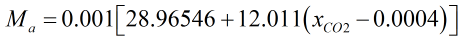Where xCO2 = Carbon dioxide concentration

### Mole Fraction of Water Vapour

The mole fraction of water vapour is calculated from the formulae below. Use the appropriate one depending on the inputs available i.e. use equation on the far right if using dew point temperature as an input.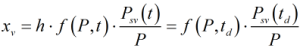Where h = Relative humidity P = Pressure Psv = Saturated vapour pressure of moist air f = Enhancement factor

### Saturated Vapour Pressure of Moist Air

The vapour pressure at saturation, Psv is calculated from: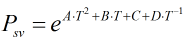Where T = Temperature in Kelvin A = 1.2378847 x 10-5 K-2 B = 1.9121316 x 10-2 K-1 C = 33.93711047 D = -6.3431645 x 103 K

### Enhancement Factor

The enhancement factor, f, is calculated from: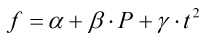Where t = Temperature in °C α = 1.0062 β = 3.14 x 10-8 Pa -1 γ = 5.6 x 10-7 K-2

### Compressibility

The compressibility, Z, is calculated from: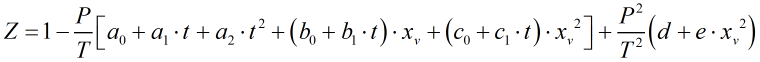Where a0 = 1.58123 x 10-6 K Pa-1 a1 = -2.9331 x 10-8 Pa-1 a2 = 1.1043 x 10-10 K-1 Pa-1 b0 = 5.707 x 10-6 K Pa-1 b1 = -2.051 x 10-8 Pa-1 c0 = 1.9898 x 10-4 K Pa-1 c1 = -2.376 x 10-6 Pa-1 d = 1.83 x 10-11 K2 Pa-2 e = -0.765 x 10-8 K2 Pa-2

### Density of Moist Air

The density of moist air, ρa is calculated from: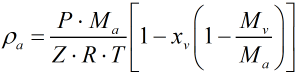Back